Next: Euler angles Up: Rigid body rotation Previous: Principal axes of rotation

# Euler's equations

The fundamental equation of motion of a rotating body [see Equation (8.3)],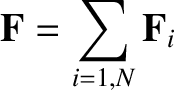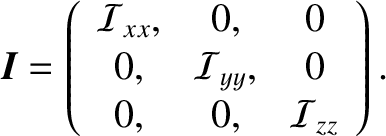(8.23)

is only valid in an inertial frame. However, we have seen that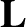is most simply expressed in a frame of reference whose axes are aligned along the principal axes of rotation of the body. Such a frame of reference rotates with the body, and is, therefore, non-inertial. Thus, it is helpful to define two Cartesian coordinate systems with the same origins. The first, with coordinates,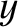,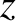, is a fixed inertial frame; let us denote this the fixed frame. The second, with coordinates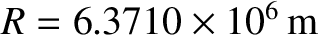,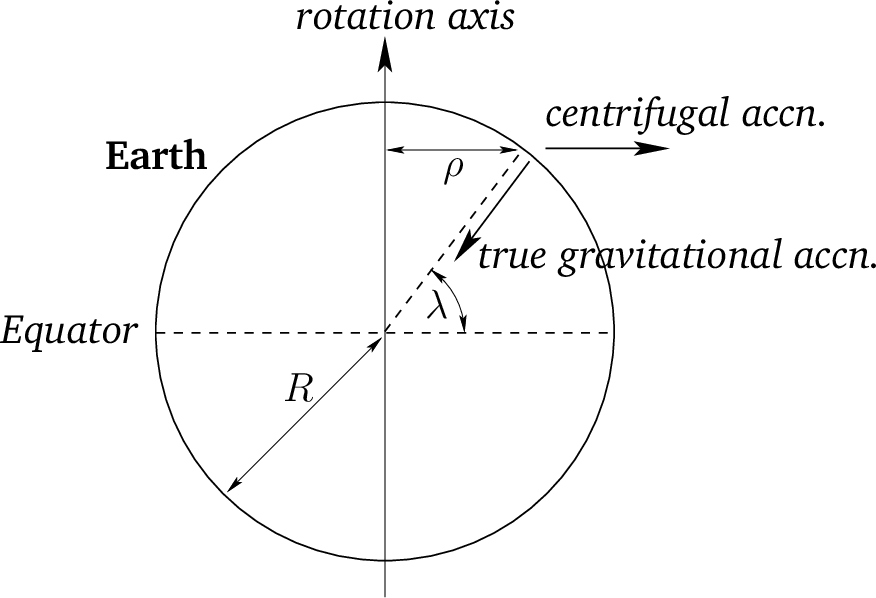,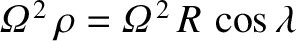, co-rotates with the body in such a manner that the-,-, and-axes are always pointing along its principal axes of rotation; we shall refer to this as the body frame. Because the body frame co-rotates with the body, its instantaneous angular velocity is the same as that of the body. Hence, it follows from the analysis in Section 6.2 that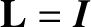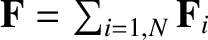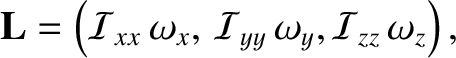(8.24)

Here,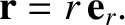is the time derivative in the fixed frame, and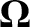the time derivative in the body frame. Combining Equations (8.23) and (8.24), we obtain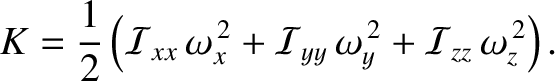(8.25)

In the body frame, let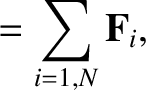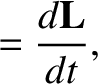and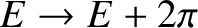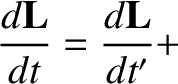. It follows that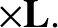(8.26)

where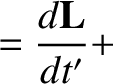,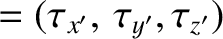and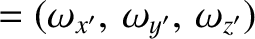are the principal moments of inertia. Hence, in the body frame, the components of Equation (8.25) yield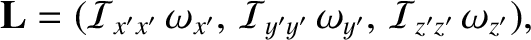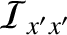(8.27)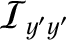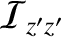(8.28) and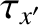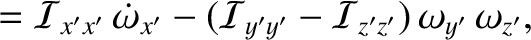(8.29)

where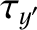. Here, we have made use of the fact that the moments of inertia of a rigid body are constant in time in the co-rotating body frame. The preceding three equations are known as Euler's equations.

Consider a body that is freely rotating; that is, in the absence of external torques. Furthermore, let the body be rotationally symmetric about the-axis. It follows that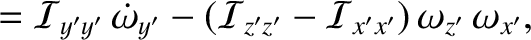. Likewise, we can write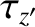. In general, however,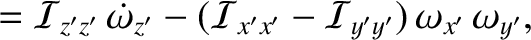. Thus, Euler's equations yield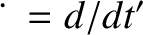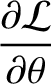(8.30)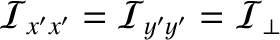(8.31) and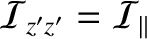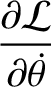(8.32)

Clearly,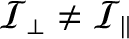is a constant of the motion. Equation (8.30) and (8.31) can be written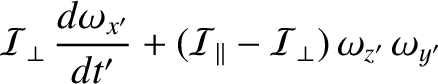(8.33) and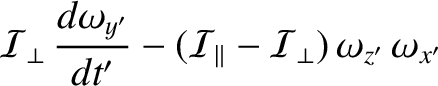(8.34)

where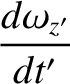. As is easily demonstrated, the solution to these equations is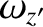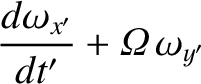(8.35) and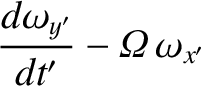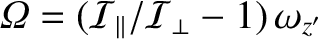(8.36)

where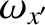is a constant. Thus, the projection of the angular velocity vector onto the-plane has the fixed length, and rotates steadily about the-axis with angular velocity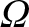. It follows that the length of the angular velocity vector,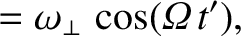, is a constant of the motion. Clearly, the angular velocity vector subtends some constant angle,, with the-axis, which implies that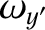and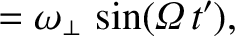. Hence, the components of the angular velocity vector are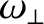(8.37)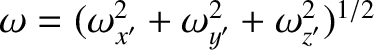(8.38) and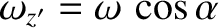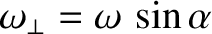(8.39)

where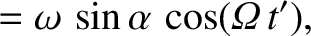(8.40)

We conclude that, in the body frame, the angular velocity vector precesses about the symmetry axis (i.e., the-axis) with the angular frequency. Now, the components of the angular momentum vector are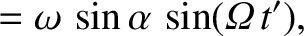(8.41)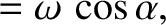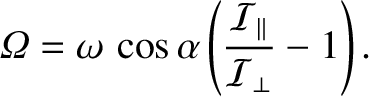(8.42) and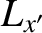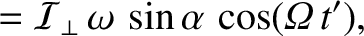(8.43)

Thus, in the body frame, the angular momentum vector is also of constant length, and precesses about the symmetry axis with the angular frequency. Furthermore, the angular momentum vector subtends a constant angle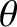with the symmetry axis, where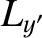(8.44)

The angular momentum vector, the angular velocity vector, and the symmetry axis all lie in the same plane; that is,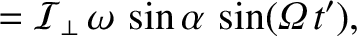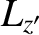, as can easily be verified. Moreover, the angular momentum vector lies between the angular velocity vector and the symmetry axis (i.e.,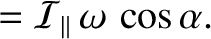) for a flattened (or oblate) body (i.e.,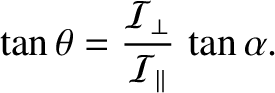), whereas the angular velocity vector lies between the angular momentum vector and the symmetry axis (i.e.,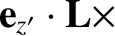) for an elongated (or prolate) body (i.e.,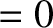). (See Figure 8.2.)Next: Euler angles Up: Rigid body rotation Previous: Principal axes of rotation
Richard Fitzpatrick 2016-03-31